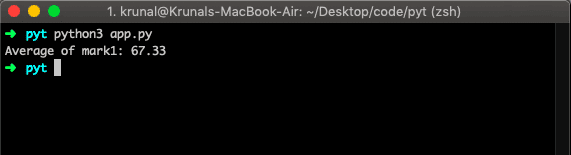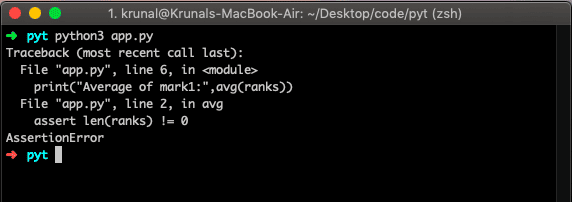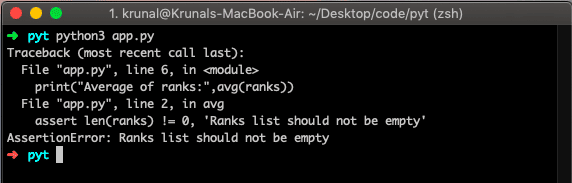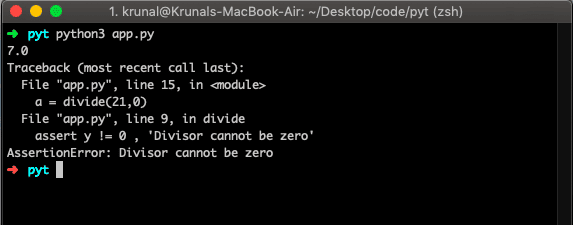# Python assert: The Complete Guide

If you want to simulate or debug your code, like what is happening in which stage, then you can use the python assert statements in your code.

It is a debugging tool as it stops a program as soon as any error occurs and shows at which point of the program that error has occurred.

Let’s take a simple example of writing the division function; you’re confident that the divisor shouldn’t be zero, and you assert divisor is not equal to zero. We will see this example later in this post.

## What is Assertion

Assertions are statements that assert or state a case confidently in your program. For example, assertions in Python are boolean expressions that check if the conditions return true or not. If true, the program does nothing and continues executing the next line of code.

However, if it’s False, the program stops and throws an error.

### Syntax

The following syntax is the basic structure of python assert statements.

`assert condition`

If you wish to write a message when the condition is false, then its syntax is the following.

`assert condition, message`

You can also send information like a message with the assert statement to better understand the code’s fault.

## Python assert

Python assert is built-in statements are boolean expressions to check if the condition is True.  Assertions are statements that state the fact confidently in your program.

An assertion is the sanity check that you can turn on or off when you are done with your program testing.

Assertions are the conditions or boolean expressions that are always supposed to be true in the code.

The assert statement takes an expression and an optional message.

An assert statement is used to check the types, values of the argument, and output of the function.

The assert statement is used as the debugging tool as it halts the program at the point where an error occurs.

See the following program of assertions in Python.

```# app.py

def avg(ranks):
assert len(ranks) != 0
return round(sum(ranks)/len(ranks), 2)

ranks = [62, 65, 75]
print("Average of mark1:",avg(ranks))```

In the above example, we do not want to pass the empty ranks; if it is empty, it will throw an Assertion Error. See the below output.In the above example, we will get an average because we have passed the list of items.

Now, let’s pass the empty list and see the output.

```# app.py

def avg(ranks):
assert len(ranks) != 0
return round(sum(ranks)/len(ranks), 2)

ranks = []
print("Average of mark1:",avg(ranks))```

Now, in the above example, we have not passed the rank items. So the length will be 0, and we will get an Assertion Error.If the assertion fails, Python uses ArgumentExpression as the argument for the AssertionError.

AssertionError exceptions can be caught and handled like any other exception using the try-except statement, but if not handled properly, they will terminate the program and produce the traceback.

But, the above example does not throw an error with a proper message. You can write your custom error message as well. Let’s do that.

```# app.py

def avg(ranks):
assert len(ranks) != 0, 'Ranks list should not be empty'
return round(sum(ranks)/len(ranks), 2)

ranks = []
print("Average of ranks:",avg(ranks))```

In the above example, we have passed the second argument to the assert statement, which is the message. So in the output, we will see the message as well.## Python Assert Keyword withassert with an error message

Let’s see another example which is divided by 0.

```# app.py

def divide(x, y):
assert y != 0 , 'Divisor cannot be zero'
return round(x/y, 2)

z = divide(21,3)
print(z)

a = divide(21,0)
print(a)```

In the above example, if the divisor is 0, the assert will throw an error with a message. Let’s see the output.In the third line of the above code, we can see the assert statement.

In this line, it is checked whether the variable y value is equal to 0 or not.

If it is greater than zero, i.e., the condition is true, then no problem occurs, and we will get the output accordingly.

But when we call the method division() with the 2nd argument 0, the assert condition is false.

That is why an AssertionError occurs, and it throws an error with the message ‘Divisor cannot be zero,’ that we wrote in the message part of the python assert statement.

## Python Assert Methods

Method Checks that New in
`assertEqual(x, y)` `x == y `
`assertNotEqual(x, y)` `x != y `
`assertTrue(x)` `bool(x) is True`
`assertFalse(x)` `bool(x) is False`
`assertIs(x, y)` `x isy ` 3.1
`assertIsNot(x, y)` `x is noty ` 3.1
`assertIsNone(x)` `x is None` 3.1
`assertIsNotNone(x)` `x is not None` 3.1
`assertIn(x, y)` `x iny ` 3.1
`assertNotIn(x, y)` `x not y ` 3.1
`assertIsInstance(x, y)` `isinstance(x, y)` 3.2
`assertNotIsInstance(x,y)` `not isinstance(x, y)` 3.2

### How do you test that a Python function throws an exception

We can use `TestCase.assertRaises` (or TestCase.failUnlessRaises) from the unit test module, for example.

```# app.py

import unittest

def broken_function():
raise Exception('This is broken')

class MyTestCase(unittest.TestCase):
def test(self):
with self.assertRaises(Exception) as context:
broken_function()

self.assertTrue('This is broken' in str(context.exception))

if __name__ == '__main__':
unittest.main()
```

See the output.

```➜  pyt python3 app.py
.
----------------------------------------------------------------------
Ran 1 test in 0.000s

OK
➜  pyt
```

## Common Pitfalls With Using Asserts in Python

There are two important cautions with using assertions in Python that I’d like to shout out.

1. The first one concerns introducing security risks and bugs into your applications. Don’t Use Asserts for Data Validation.
2. The second one is about a syntax quirk that makes it easy to write useless assertions. Asserts That Never Fail.

## Key Points of Assert In Python

1. Assertions are the condition or boolean expressions which are always supposed to be true in the code.
2. The assert statement takes an expression and an optional message.
3. The assert statement is used to check the types, values of the argument, and output of the function.
4. The assert statement is used as a debugging tool as it halts the program at the point where an error occurs.
5. The proper use of assertions is to inform developers about unrecoverable errors in a program. They’re not intended to signal expected the error conditions, like “file not found”, where the user can take corrective action or try again.
6. Assertions are internal self-checks for your program. They work by declaring some conditions as impossible in your code. If one of these conditions doesn’t meet, your program has a bug.

That’s it for this tutorial.

## Recommended Posts

Python Try Except

Python iter

Python float

Null Object in Python

Python pow()

This site uses Akismet to reduce spam. Learn how your comment data is processed.